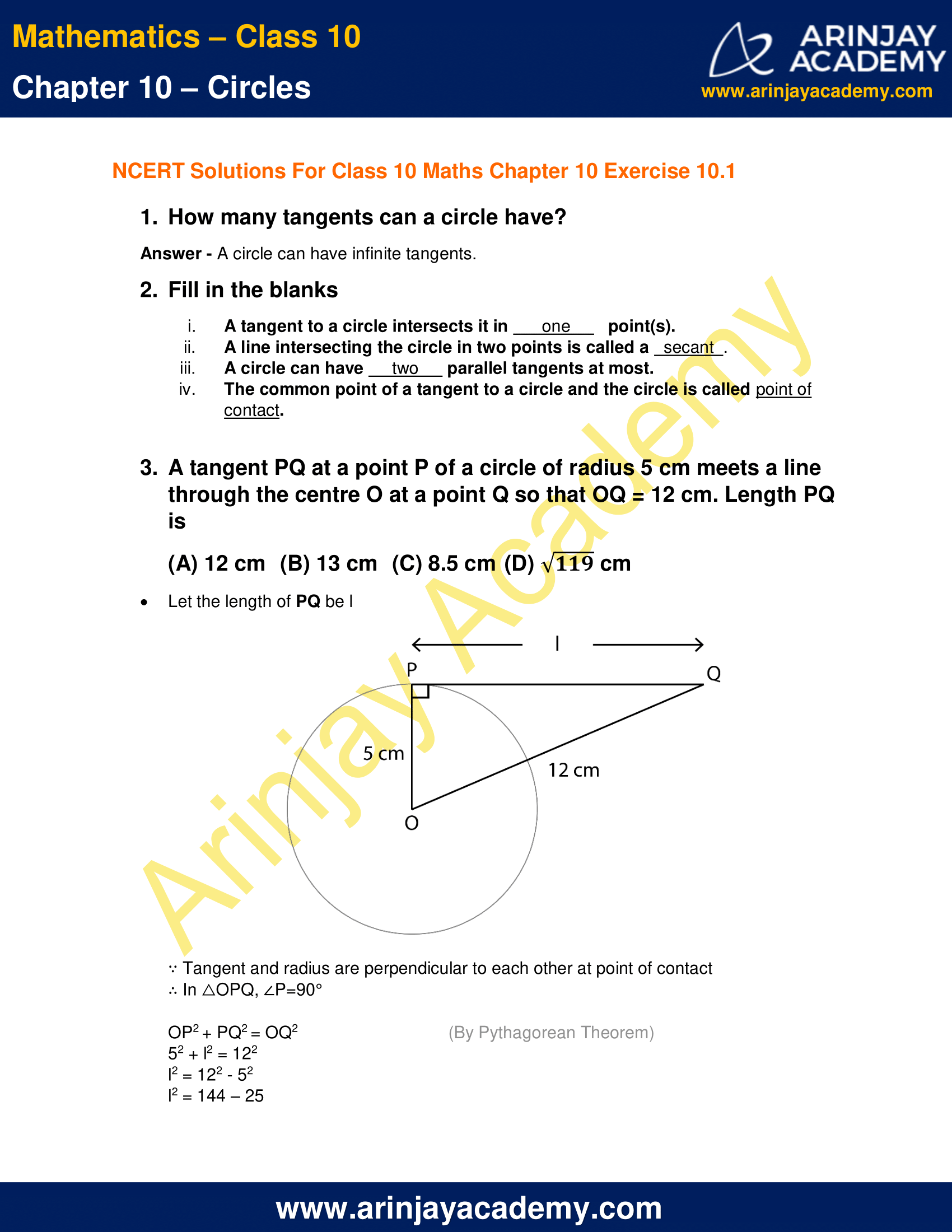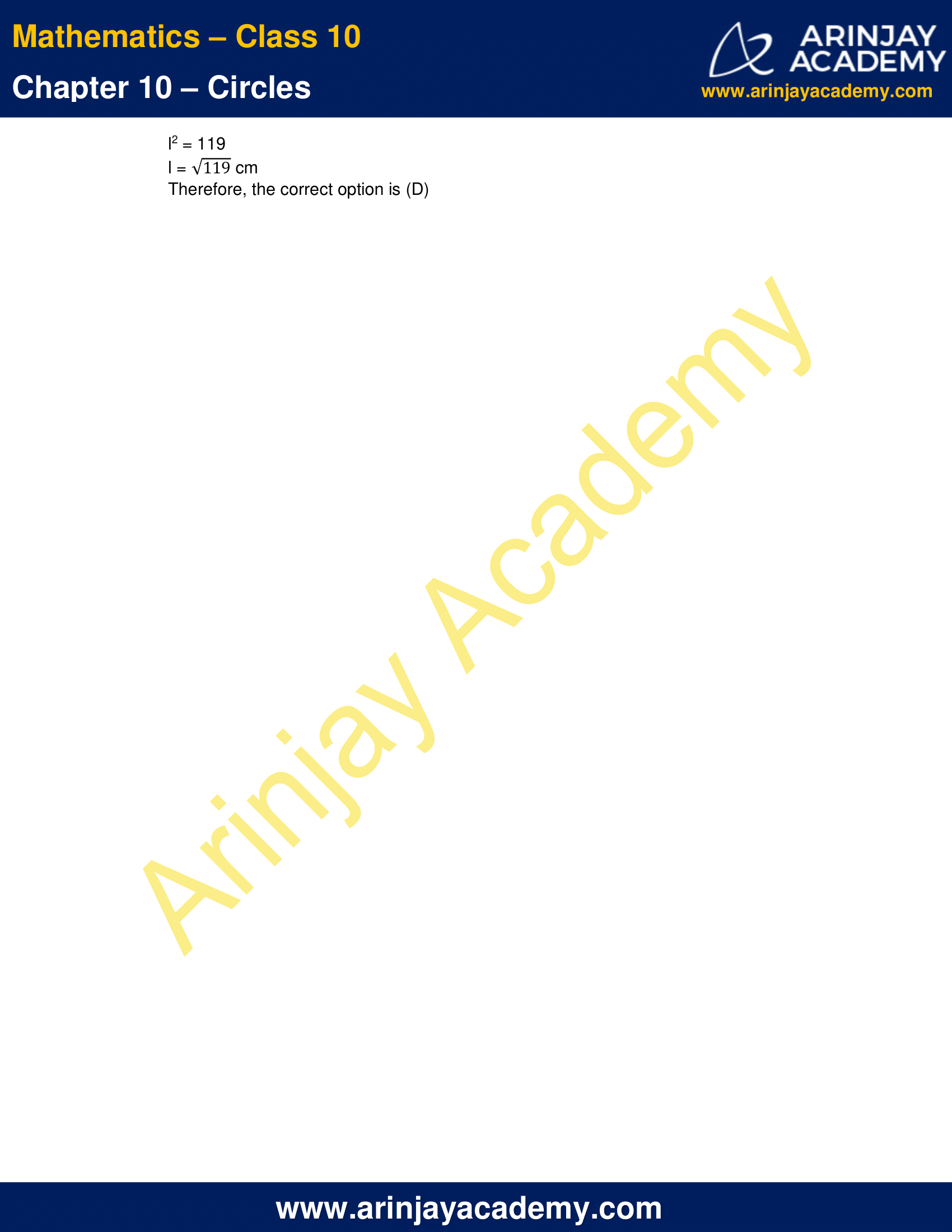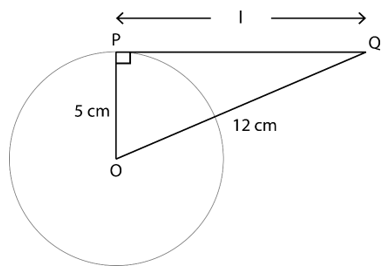# NCERT Solutions For Class 10 Maths Chapter 10 Exercise 10.1 – Circles

Download NCERT Solutions For Class 10 Maths Chapter 10 Exercise 10.1 – Circles. This Exercise contains 3 questions, for which detailed answers have been provided in this note. In case you are looking at studying the remaining Exercise for Class 10 for Maths NCERT solutions for other Chapters, you can click the link at the end of this Note.

### NCERT Solutions For Class 10 Maths Chapter 10 Exercise 10.1 – CirclesNCERT Solutions For Class 10 Maths Chapter 10 Exercise 10.1 – Circles

1. How many tangents can a circle have?

Answer – A circle can have infinite tangents.

2. Fill in the blanks

i. A tangent to a circle intersects it in one  point(s).
ii. A line intersecting the circle in two points is called a secant  .
iii. A circle can have two  parallel tangents at most.
iv. The common point of a tangent to a circle and the circle is called point of contact.

3. A tangent PQ at a point P of a circle of radius 5 cm meets a line through the centre O at a point Q so that OQ = 12 cm. Length PQ is

(A) 12 cm  (B) 13 cm  (C) 8.5 cm (D) √119 cm

Let the length of PQ be l∵ Tangent and radius are perpendicular to each other at point of contact
∴ In △OPQ, ∠P=90°
OP2 + PQ2 = OQ2 (By Pythagorean Theorem)
52 + l2 = 122
l2 = 122 – 52
l2 = 144 – 25
l2 = 119
l = √119 cm
Therefore, the correct option is (D)

NCERT Solutions for Class 10 Maths Chapter 10 Exercise 10.1 – Circles, has been designed by the NCERT to test the knowledge of the student on the topic – Tangent to a Circle

Download NCERT Solutions For Class 10 Maths Chapter 10 Exercise 10.1 – Circles